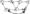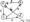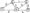# Analysis and Design of Algorithms Jan 2007

Note : Answer any FIVE full questions.

1  a. What is an Algorithm? Illustrate the important points to be noted with respect to an algorithm, with an example.

b.  Describe the standard algorithm for finding the binary representation of a positive decimal integer with a neat pseudo code.

c.   Design an algorithm for checking whether two given words are anagrams, for example the words ‘tea’ and ‘eat’ are anagrams, i.e. one word can be obtained by permuting the letters of the other.

2 a. For the following algorithms, indicate i) Natural size metric for its inputs ii) Basic operations iii) Whether the basic operations count can be different for inputs of the same size.

1) Computing sum of £n’numbers

2) Computing x11.

3) Finding largest element in a list of ‘n’ numbers.

4) Euclid’s algorithm.

b.  Find the Big – on notation for the following :

i) log n + -\fn ii) n + n log n iii) 6n + 2n4 + 4n5

c.   Find the time efficiency of the definition based algorithm for computing product of n x n matrices.

4 a. Design a brute – force algorithm for computing the value of a polynomial p(x)=ax“+a„-i + avx + aQ at a given ‘xo’ and determine it’s worst case efficiency class.

b.  Sort the list { E, X, A, M, P, L, E} in alphabetical order using insertion sort.

c.   Give the pseudo code for finding maximum and minimum element in an array of ‘n’ numbers using divide – and – conquer technique.

5 a. Draw the tree of recursive calls made to sort the elements { C,0, M, P, U, T, I, N, G } in alphabetical order using Quick sort method.

b.  Apply Strassen’s algorithm to compute, using 2×2 matrices, exiting the recursion when n-2.

 “1 0 2 f ~0 1 0 1“ 4 1 1 0 * 2 1′ 0 4 0 1 3 0 2 0 1 1 5 0 2 1_ 1 3 5 0_

C. Apply the D F S – based and source removal methods to obtain the topological sorting of the following graph.Fig.5(c)

6 (a)Construct the AVL tree and 2-3 tree for the input sequence : 3 6 5 1 2 4

(b) Sort the elements { S, O, R, T, I, N, G } in alphabetical order using Heapsort method. Construct the open hash table and closed hash table for the input:

30^ 20, 56, 75, 31, 19 using the hash function h (k) = k mod 11

7 (a) Apply Floyd’s algorithm to compute all – pairs shortest paths for the following graph.(b) Apply KruscaTs algorithm to find a minimum spanning tree of the following g ph.Fig. 7(b)

8 (a) Construct a Huffman code for the following data.

 Character A B C D Probability 0.4 0.1 0.2 0.15 0.15

(b)Prove that the classic recursive algorithm for the Tower of Hanoi problem makes the smallest member of disk moves needed to solve the problem.

(c)Draw the state – space tree for solving 4-Queens problem using back tracking.

(d) Solve the following instance of the Knapsack problem by the branch – and – bound algorithm.

 Item Weight Value 1 10 \$ 100 2 7 \$63 3 8 \$56 4 4 \$ 12